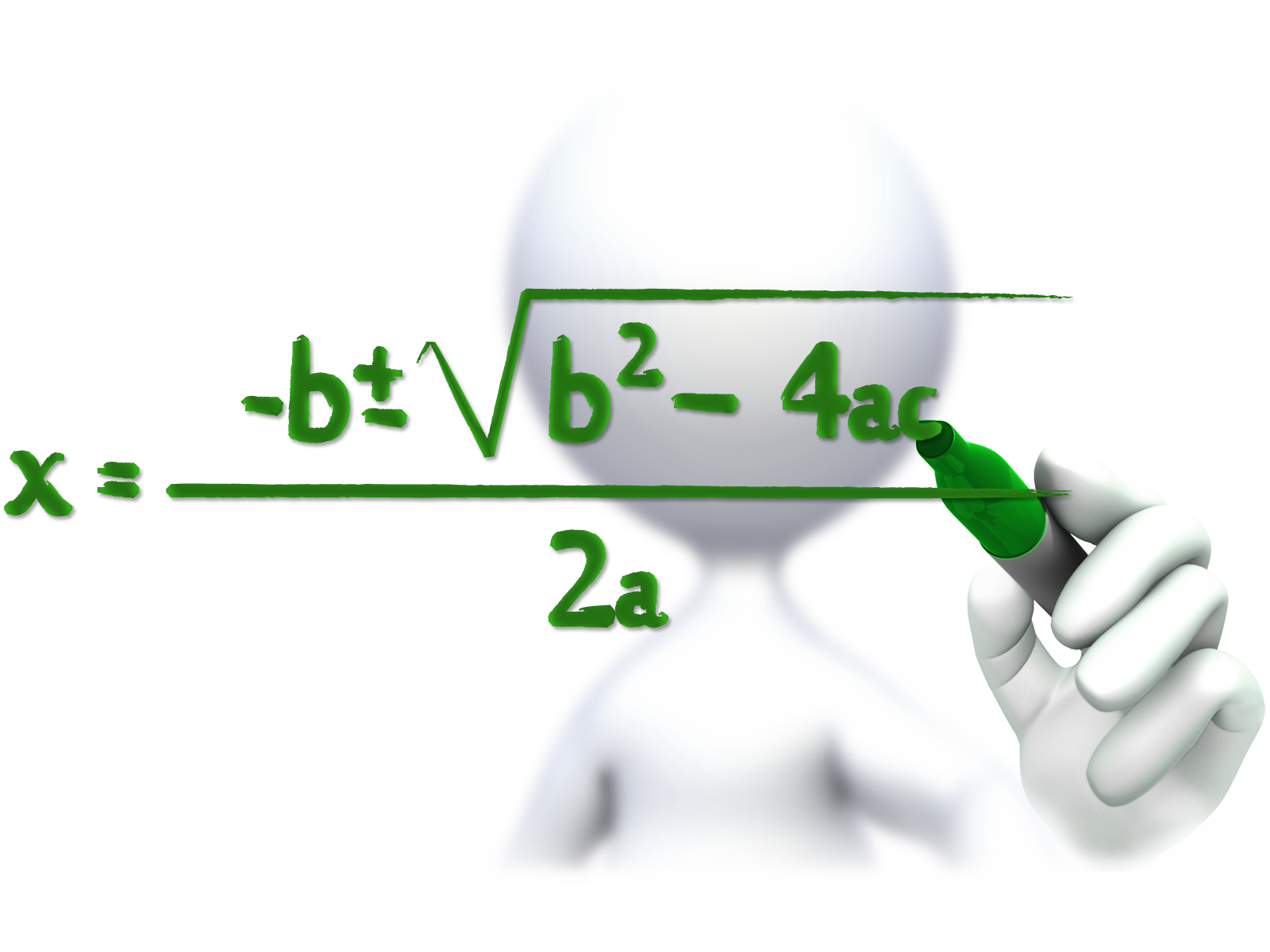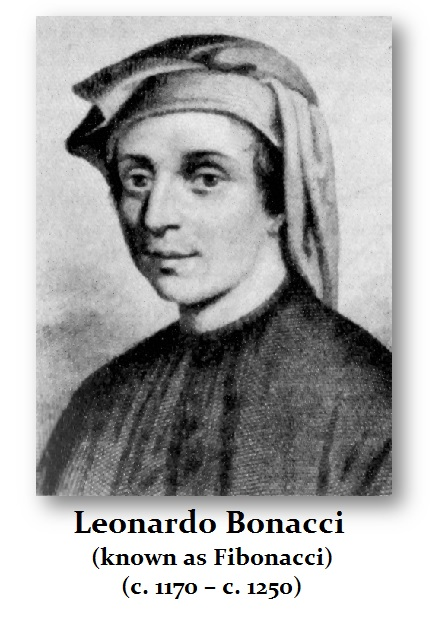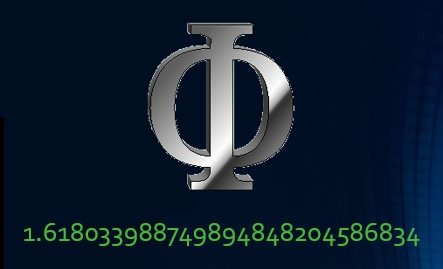# Mathematics & Dark AgeWe tend to judge far too much by our own times. What cannot be overlooked is the lack of mathematics following the Dark Ages. When numbers were Roman numerals, calculation and bookkeeping were not supportive of international accounting. Interestingly enough, as pornography enabled video streaming, it was gambling that gave birth to mathematics. Vices often inspire innovation.For example, the 1202 publication of “Liber Abaci” (Book of Abacus) by Leonardo Pisano or Bonacci of Pisa , known to most as Fibonacci (1170-1240 AD). When Fibonacci published his “Liber Abaci”, it introduced Hindu-Arabic numerals. This method allowed calculation that was not taught in schools and was unknown in Christian circles, except among a very small group of intellectuals who had access to translations ofthe Arab mathematician al-Khwarizmi (780-850 AD).

Fibonacci’s “Liber Abaci” introduced vital concepts that enable banking to emerge. The first seven chapters dealt with notation’s introduction of the idea of a place value, whereas the position of a figure determined where it is a unit, such as 10, 100, 1000 and so on. He also introduced the use of numerals in arithmetical calculations. These techniques were groundbreaking for a culture that lost its identity with the fall of Rome. Fibonacci illustrates practical problems on how to calculate profit margin, moneychanging, barter, conversion of weights and measures, partnerships, and last but not least, interest. He even introduced some geometry and algebra. This work was so earth-shattering, it became the topic of discussion and caught the attention of Holy Roman Emperor Frederick II (1215-1250 AD)(King of Sicily 1198-1250 AD).Fibonacci was given a test; the third problem  was x3+2×2+10x=20 (modern notation). He solved the equation using the Babylonian method based upon sexagesimal fractions (base 60), which when translated into modern decimals is 1.3688081075. Fibonacci is strangely best known for his sequence derived from a pair of multiplying rabbits (1, I, 2, 3, 5, 8, 13, 21, 34, 55 .•. ) where the progression follows what has become known as the “golden ratio” 1.6180 that was discovered by the University of Glascow mathematician Robert Simson in 1753.

The Arabs and the Jews were always highly educated and were not victims of the superstitions of the times. Thus, the knowledge of mathematics was highly restricted to these groups back then. Jews were not just moneylenders, but they had also been captains of ships where Christians were ignorant of mathematics. Therefore, Fibonacci opened the door for capitalism by first creating a knowledge base of mathematics by introducing the decimal system to Europe. Finally, the secrets of mathematics were starting to resurface from the Dark Ages.

Knowledge seems to evaporate when going into a Dark Age. The question remains, what knowledge will evaporate this time if we go back that far?